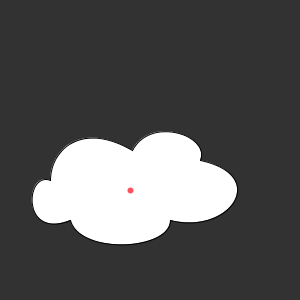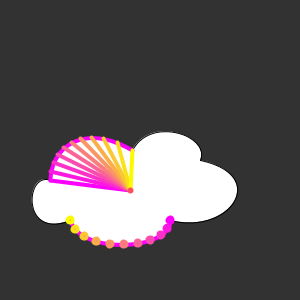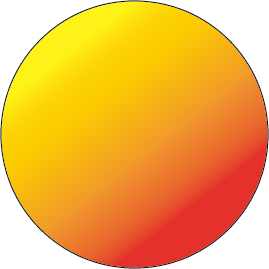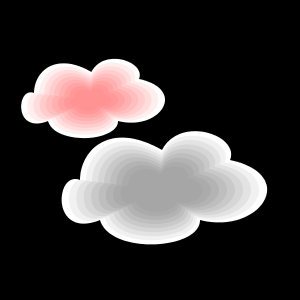#### Howdy, Stranger!

We are about to switch to a new forum software. Until then we have removed the registration on this forum.

# In the Bezier curve, how to make it from the center point to the surrounding color gradient?

edited April 2018

Hello Processing Community, I have created a sketch ,But in the Beziercurve, how to make it from the center point to the surrounding color gradient? Until fill the entire graph.how to use Processing to achieve it? waiting for your suggestions and trying my best for this... regards ...

``````    //color from = color (232, 255, 62);
//color to = color (255, 62, 143);
//int j=4;

void setup(){
size(300, 300);
background(50);
smooth();
}
void draw(){

//for (int i=1;i<j;i++) {
//   color interA=lerpColor(from, to, (float(i)/j));
//   fill(interA);
fill(255);
beginShape();
vertex(50, 180);
bezierVertex(50, 150, 80, 120, 132, 150);
bezierVertex(150, 115, 210, 135, 200, 160);
bezierVertex(270, 175, 230, 235, 170, 220);
bezierVertex(170, 250, 80, 255, 70, 220);
bezierVertex(20, 240, 25, 170, 50, 180);
endShape();

//center
stroke(#ff5060);
strokeWeight(6);
point(130,190);
noLoop();

}
``````
Tagged:

• ok, so currently it looks like this:what do you want it to look like?

Do you want the gradient to be on the background, or on the cloud?

• I want it to change from the center of the circle to the color of the cloud，a radial color gradient.

• Demo below. I demo how to draw points on a curve and then a second demo how to color from the center to any of those points.

A second approach is to create the radial color in the background and use the cloud shape as a mask, similar to a cookie-cutter.

Kf``````final color STROKECOLOR1=color(250, 0, 250);
final color STROKECOLOR2=color(250, 250, 0);

float p1x;
float p1y;
float p2x;
float p2y;

float c1x;
float c1y;
float c2x;
float c2y;

PVector centroid;

void setup() {
size(300, 300);
background(50);
smooth();

centroid = new PVector(130, 190);
}
void draw() {

//for (int i=1;i<j;i++) {
//   color interA=lerpColor(from, to, (float(i)/j));
//   fill(interA);
fill(255);
beginShape();
vertex(50, 180);
bezierVertex(50, 150, 80, 120, 132, 150);
bezierVertex(150, 115, 210, 135, 200, 160);
bezierVertex(270, 175, 230, 235, 170, 220);
bezierVertex(170, 250, 80, 255, 70, 220);
bezierVertex(20, 240, 25, 170, 50, 180);
endShape();

stroke(255, 0, 250);
strokeWeight(4);
noFill();

setGlobalPoints(50, 180, 50, 150, 80, 120, 132, 150);
drawBezier(STROKECOLOR1);
drawPointToBezier(10,STROKECOLOR1,STROKECOLOR2,centroid);

setGlobalPoints(170, 220,170, 250, 80, 255, 70, 220);
drawBezier(STROKECOLOR1);
drawBezierPoints(10,STROKECOLOR1,STROKECOLOR2);

//center
stroke(#ff5060);
strokeWeight(6);
point(centroid.x,centroid.y);
noLoop();
}

void drawBezierPoints(int steps, color cs1,color cs2) {
for (int i = 0; i <= steps; i++) {
float t = i / float(steps);
float x = bezierPoint(p1x, c1x, c2x, p2x, t);
float y = bezierPoint(p1y, c1y, c2y, p2y, t);
stroke(lerpColor(cs1,cs2,t));
ellipse(x, y, 5, 5);
}
}

void drawPointToBezier(int steps, color cs1,color cs2, PVector centroid) {
for (int i = 0; i <= steps; i++) {
float t = i / float(steps);
float x = bezierPoint(p1x, c1x, c2x, p2x, t);
float y = bezierPoint(p1y, c1y, c2y, p2y, t);
stroke(lerpColor(cs1,cs2,t));
line(centroid.x,centroid.y, x, y);
}
}

void setGlobalPoints(float... a) {
p1x=a;
p1y=a;
c1x=a;
c1y=a;

p2x=a;
p2y=a;
c2x=a;
c2y=a;
}

void drawBezier(color cs) {
stroke(cs);
noFill();
bezier(p1x, p1y, c1x, c1y, c2x, c2y, p2x, p2y);
}
``````
• I want it to change from the center of the circle to the color of the cloud，a radial color gradient.

draw us a picture

• I want to be like this, this circle is the cloud, fill the radial gradient color outward from the center point of the cloud, the color is given in the above code.• One way to do it is to modify the data so that the cloud data is centred about the point [0,0] you can then draw the shape many times with different colours at different scales to give the illusion of a flood fill from the center. The shape is then translated to where you want the cloud to be drawn.

Using a separate function to draw 'a cloud' makes it possible to draw multiple clouds of different sizes and colours.

The code below used this approach to produce this image -This image was created with the code below.

``````void setup() {
size(300, 300);
background(50);
smooth();
}

void draw() {
background(0);
drawCloud(90, 100, 0.2, 0.7, color(255), color(255, 100, 100, 32));
drawCloud(160, 190, 0.4, 1.0, color(255), color(128, 32));
noLoop();
}

/**
Parameters
px, py pixel position of cloud centre
s0 scale of the inner cload
s1 scale of the full cloud (Note s1 > s0)
c0 = colour at the clod edge
c1 = colour at the clode centre
*/
void drawCloud(float px, float py, float s0, float s1, int c0, int c1) {
int nbrSteps = 8;
float deltaScale = (s1 - s0) / nbrSteps;
float deltaT = 1.0 / nbrSteps;
noStroke();
pushMatrix();
translate(px, py);
for (int n = 0; n <= nbrSteps; n++) {
fill(lerpColor(c0, c1, n * deltaT));
pushMatrix();
scale(s1 - n * deltaScale);
beginShape();
vertex(-80, -10);
bezierVertex(-80, -40, -50, -70, 2, -40);
bezierVertex(20, -75, 80, -55, 70, -30);
bezierVertex(140, -15, 100, 45, 40, 30);
bezierVertex(40, 60, -50, 65, -60, 30);
bezierVertex(-110, 50, -105, -20, -80, -10);
endShape();
popMatrix();
}
popMatrix();
}
``````
• I do not quite understand how your bezierVertex coordinate values are calculated? Can you read it for me?

• In your original code the Bezier coordinates were actual screen coordinates and you said the cloud centre was at [130, 190]. To get my Bezier points I have subtracted 130 from the X value and 190 from the Y value so the cloud centre is now [0,0].

The cloud can be drawn anywhere now by translating the axis (line 28) before drawing